User:Tohline/Apps/MaclaurinSpheroids

(diff) ← Older revision | Current revision (diff) | Newer revision → (diff)

Maclaurin Spheroids (axisymmetric structure)

 |   Tiled Menu   |   Tables of Content   |  Banner Video   |  Tohline Home Page   |

There is no particular reason why one should guess ahead of time that the equilibrium properties of any rotating, self-gravitating configuration should be describable in terms of analytic functions. As luck would have it, however, the gravitational potential at the surface of and inside an homogeneous spheroid is expressible analytically. (The potential is constant on concentric spheroidal surfaces that generally have a different axis ratio from the spheroidal mass distribution.) Furthermore, the gradient of the gravitational potential is separable in cylindrical coordinates, proving to be a simple linear function of both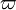$\varpi$ and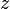$~z$.

If the spheroid is uniformly rotating, this behavior conspires nicely with the behavior of the centrifugal acceleration — which also will be a linear function of$\varpi$ — to permit an analytic (and integrable) prescription of the pressure gradient. Not surprisingly, it resembles the functional form of the pressure gradient that is required to balance the gravitational force in uniform-density spheres.

As a consequence of this good fortune, the equilibrium structure of a uniformly rotating, uniform-density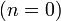$~(n = 0)$, axisymmetric configuration can be shown to be precisely an oblate spheroid whose internal properties are describable in terms of analytic expressions. As we show in an accompanying discussion, the angular velocity that is required to keep a self-gravitating spheroid of a specified eccentricity in equilibrium can be obtained from a theorem — we will refer to it as Maclaurin's Theorem — that was derived from purely geometric arguments over 270 years ago by Colin Maclaurin (1742) in A Treatise of Fluxions. This result has been enumerated in many subsequent publications (e.g., Tassoul 1978; Chandrasekhar 1987). It should be appreciated that both Volume I and Volume II of Maclaurin's (1742) Treatise can now be accessed online via Google Books. Selected excerpts from these two volumes are shown in our accompanying discussion.

Properties of Uniform-Density Spheroids

Surface Definition

Let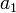$~a_1$ be the equatorial radius and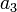$~a_3$ the polar radius of a uniform-density object whose surface is defined precisely by an oblate spheroid. The degree of flattening of the object may be parameterized in terms of the axis ratio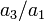$~a_3/a_1$, or in terms of the object's eccentricity,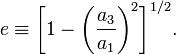$e \equiv \biggl[ 1 - \biggl(\frac{a_3}{a_1}\biggr)^2 \biggr]^{1/2} .$

(For an oblate spheroid,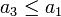$~a_3 \leq a_1$; hence, the eccentricity is restricted to the range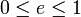$~0 \leq e \leq 1$.) The meridional cross-section of such a spheroid is an ellipse with the same eccentricity. The foci of this ellipse lie in the equatorial plane of the spheroid at a distance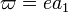$~\varpi = ea_1$ from the minor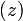$~(z)$ axis.

For purposes of normalization, it will be useful to define the mean radius of the spheroid as,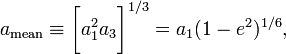$~a_\mathrm{mean} \equiv \biggl[a_1^2 a_3 \biggr]^{1/3} = a_1 (1 - e^2)^{1/6} ,$

which is equivalent to the radius of a sphere in the limit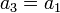$~a_3 = a_1$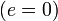$~(e=0)$.

Mass

The total mass of such a spheroid is,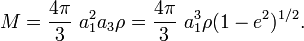$M = \frac{4\pi}{3}~a_1^2 a_3 \rho = \frac{4\pi}{3}~a_1^3 \rho (1 - e^2)^{1/2} .$

Gravitational Potential

In an accompanying discussion entitled, Properties of Homogeneous Ellipsoids, an expression is given for the gravitational potential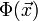$\Phi(\vec{x})$ at an internal point or on the surface of an homogeneous ellipsoid with semi-axes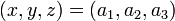$~(x,y,z) = (a_1,a_2,a_3)$. For an homogeneous, oblate spheroid in which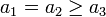$~a_1 = a_2 \geq a_3$, this analytic expression defining the potential reduces to the form,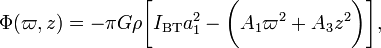$\Phi(\varpi,z) = -\pi G \rho \biggl[ I_\mathrm{BT} a_1^2 - \biggl(A_1 \varpi^2 + A_3 z^2 \biggr) \biggr],$

where, the coefficients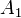$~A_1$,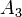$~A_3$, and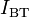$~I_\mathrm{BT}$ are functions only of the spheroid's eccentricity. Specifically,$~A_1$$~=$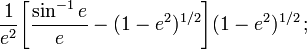$\frac{1}{e^2} \biggl[\frac{\sin^{-1}e}{e} - (1-e^2)^{1/2} \biggr](1-e^2)^{1/2} \, ;$$~A_3$$~=$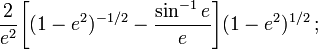$\frac{2}{e^2} \biggl[(1-e^2)^{-1/2} -\frac{\sin^{-1}e}{e} \biggr](1-e^2)^{1/2} \, ;$$~I_\mathrm{BT}$$~=$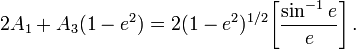$~2A_1 + A_3(1-e^2) = 2 (1-e^2)^{1/2} \biggl[ \frac{\sin^{-1}e}{e}\biggr] \, .$

Note that these three expressions have the following values in the limit of a sphere$~(e=0)$ or in the limit of an infinitesimally thin disk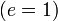$~(e=1)$:

 Limiting Values (for oblate spheroids)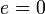$~e=0$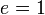$~e=1$$~A_1$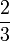$\frac{2}{3}$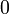$~0$$~A_3$$\frac{2}{3}$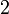$~2$$~I_\mathrm{BT}$$~2$$~0$

Equilibrium Structure

Governing Relations

To obtain the equilibrium structure of Maclaurin spheroids, we will adopt the technique outlined earlier for determining the structure of axisymmetric configurations. Specifically, the algebraic expression,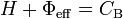$~H + \Phi_\mathrm{eff} = C_\mathrm{B}$ ,

must be solved in conjunction with the Poisson equation written in cylindrical coordinates for axisymmetric configurations, namely,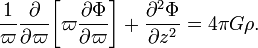$~\frac{1}{\varpi} \frac{\partial }{\partial\varpi} \biggl[ \varpi \frac{\partial \Phi}{\partial\varpi} \biggr] + \frac{\partial^2 \Phi}{\partial z^2} = 4\pi G \rho .$

Expression for Effective Potential

For any value of the eccentricity,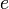$~e$, the above expression for the gravitational potential satisfies this two-dimensional Poisson equation. Furthermore, an algebraic expression defining the centrifugal potential inside a uniformly rotating configuration can be drawn from our accompanying table that summarizes the properties of various simple rotation profiles. Together, these relations give us the relevant expression for the effective potential, namely,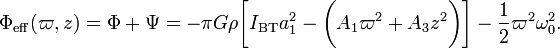$\Phi_\mathrm{eff}(\varpi,z) = \Phi + \Psi = -\pi G \rho \biggl[ I_\mathrm{BT} a_1^2 - \biggl(A_1 \varpi^2 + A_3 z^2 \biggr) \biggr] - \frac{1}{2}\varpi^2 \omega_0^2 .$

Hence, the enthalpy throughout the configuration must be given by the expression,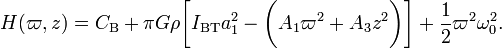$H(\varpi,z) = C_\mathrm{B} + \pi G \rho \biggl[ I_\mathrm{BT} a_1^2 - \biggl(A_1 \varpi^2 + A_3 z^2 \biggr) \biggr] + \frac{1}{2}\varpi^2 \omega_0^2 .$

This expression contains two constants,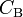$~C_\mathrm{B}$ and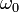$~\omega_0$, that can be determined from relevant boundary conditions.

Apply Boundary Conditions

The enthalpy should go to zero everywhere on the surface of the spheroid. By pinning the surface down at two points and setting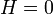$~H=0$ at both of these locations, we can determine the two unknown constants in the above expression. We choose to pin down the edge of the configuration in the equatorial plane — i.e., at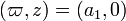$(\varpi,z) = (a_1,0)$ — and along the symmetry axis at the pole — i.e., at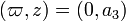$(\varpi,z) = (0,a_3)$. From the boundary condition at the pole, we derive the Bernoulli constant, specifically,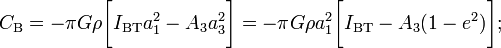$C_\mathrm{B} = - \pi G \rho \biggl[ I_\mathrm{BT} a_1^2 - A_3 a_3^2 \biggr] = - \pi G \rho a_1^2 \biggl[ I_\mathrm{BT} - A_3 (1-e^2) \biggr] ;$

and from the boundary condition in the equatorial plane we derive the rotational angular velocity, specifically,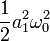$\frac{1}{2}a_1^2 \omega_0^2$$~=$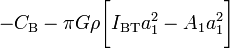$- C_\mathrm{B} - \pi G \rho \biggl[ I_\mathrm{BT} a_1^2 - A_1 a_1^2 \biggr]$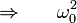$\Rightarrow ~~~~~ \omega_0^2$$~=$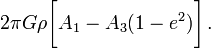$2\pi G \rho \biggl[ A_1 - A_3 (1-e^2) \biggr] \, .$

Plugging these constants into the expression for the enthalpy results in the desired solution,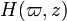$H(\varpi,z)$$~=$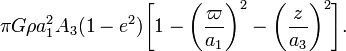$\pi G \rho a_1^2 A_3 (1-e^2)\biggl[1 - \biggl( \frac{\varpi}{a_1} \biggr)^2 - \biggl( \frac{z}{a_3} \biggr)^2 \biggr] .$

We know from our separate discussion of supplemental, barotropic equations of state that, for a uniform-density,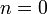$~n = 0$ polytropic configuration, the pressure is related to the enthalpy via the expression,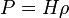$~P = H\rho$. Hence, we conclude that,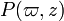$P(\varpi,z)$$~=$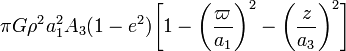$\pi G \rho^2 a_1^2 A_3 (1-e^2)\biggl[1 - \biggl( \frac{\varpi}{a_1} \biggr)^2 - \biggl( \frac{z}{a_3} \biggr)^2 \biggr]$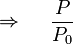$\Rightarrow ~~~~ \frac{P}{P_0}$$~=$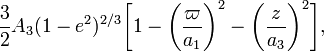$\frac{3}{2} A_3 (1-e^2)^{2/3}\biggl[1 - \biggl( \frac{\varpi}{a_1} \biggr)^2 - \biggl( \frac{z}{a_3} \biggr)^2 \biggr] ,$

where,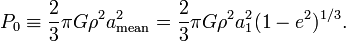$~ P_0 \equiv \frac{2}{3}\pi G \rho^2 a_\mathrm{mean}^2 = \frac{2}{3}\pi G \rho^2 a_1^2 (1-e^2)^{1/3} .$

[ T78, §4.5, Eq. (51)1 ]

Footnotes

1. From our derivation, it is clear that the leading coefficient in the pressure function$P(\varpi,z)$ can be written as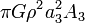$\pi G \rho^2 a_3^2 A_3$. In T78, this coefficient is given as,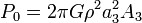$P_0 = 2\pi G\rho^2 a_3^2 A_3$, which is too large by a factor of$~2$.

Related Wikipedia Discussions

 © 2014 - 2019 by Joel E. Tohline |   H_Book Home   |   YouTube   | Context: | PGE | SR | Appendices: | Equations | Variables | References | Binary Polytropes | Ramblings | Images | Images (2016 Layout) | ADS |